Courses

# Postulates Of Quantum Mechanics MCQ Level - 2

## 10 Questions MCQ Test Topic wise Tests for IIT JAM Physics | Postulates Of Quantum Mechanics MCQ Level - 2

Description
This mock test of Postulates Of Quantum Mechanics MCQ Level - 2 for IIT JAM helps you for every IIT JAM entrance exam. This contains 10 Multiple Choice Questions for IIT JAM Postulates Of Quantum Mechanics MCQ Level - 2 (mcq) to study with solutions a complete question bank. The solved questions answers in this Postulates Of Quantum Mechanics MCQ Level - 2 quiz give you a good mix of easy questions and tough questions. IIT JAM students definitely take this Postulates Of Quantum Mechanics MCQ Level - 2 exercise for a better result in the exam. You can find other Postulates Of Quantum Mechanics MCQ Level - 2 extra questions, long questions & short questions for IIT JAM on EduRev as well by searching above.
QUESTION: 1

### Consider a set of wave functions ψi(x). Which of the following condition guarantees that the functions are normalized and mutually orthogonal? (i, j  take values from 1 to n)

Solution:

For the states to be orthogonal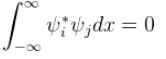and for them to be normalised,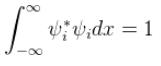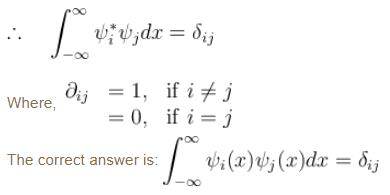QUESTION: 2

### The phase velocity of ripples on a liquid surface is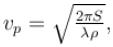where S is the surface tension and ρ the density of the liquid. The group velocity of the ripples is given by :

Solution: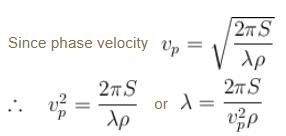As wave number,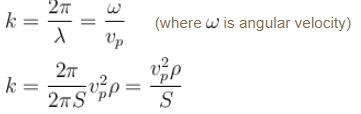We know that group velocity given by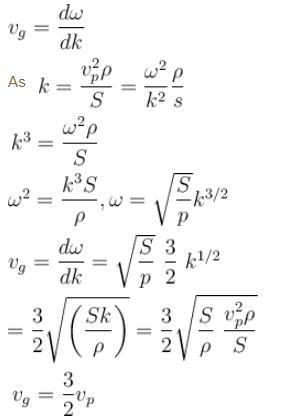The correct answer is: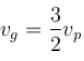QUESTION: 3

### The solutions of a free particle are :

Solution: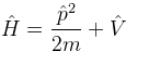V = 0 for a free particle
∴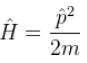Schrödinger equation.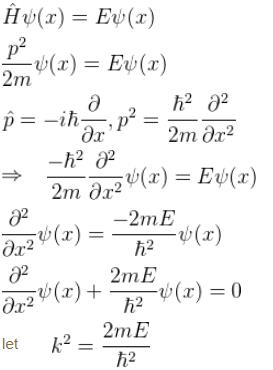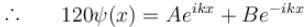(General solution)
∴    Aeikx   and  Be–ikx  are solution to the free particle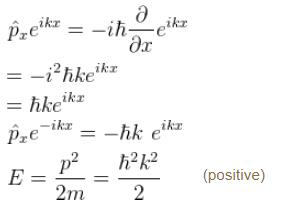The correct answer is: Energy and momentum eigen functions with both positive and negative momentum eigenvalues.

QUESTION: 4

The wave function for a particle constrained to move in 1D is shown in the graph below.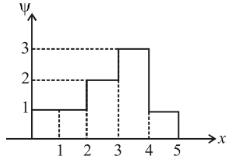What is the probability that the particle would be found between x = 2 and x = 4 ?

Solution: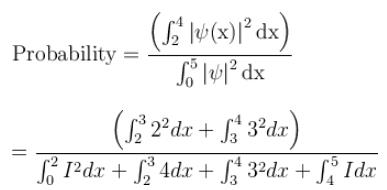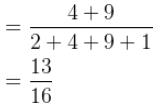QUESTION: 5

The probability current density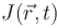is given as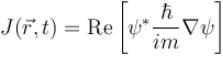Which of the following is true?

Solution:

From the continuity equation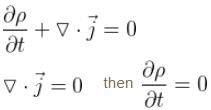i.e. p is constant in time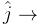Probability current density
The correct answer is: If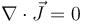then probability density is constant in time.

QUESTION: 6

Smallest possible uncertainty in position of the electron moving with velocity 3 × 107 m/s. Given, h = 6.63 × 10–34 Js, m0 = 9.1 × 10–31 kg.

Solution:

Given, v = 3 × 107 m/s
Let    Δxmin be the minimum uncertainty in position of the electron and Δp the maximum uncertainty in the momentum of the electron.

Thus, we have,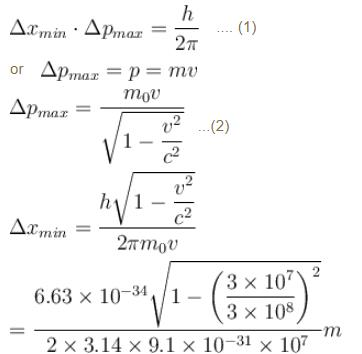= 0.03867 × 0.9949 × 10–10 m
= 3.8 × 10–12 m
The correct answer is: 3.8 × 10–12 m

QUESTION: 7

Choose the correct statement

Solution:

The correct answer is: All the energy eigenvalues are always real.

QUESTION: 8

The wave function of the particle lies in which region?

Solution:

The particle cannot exist outside the box, as it cannot have infinite amount of energy. Thus, it’s wave function is between 0 and L, where L is the length of the side of the box.

QUESTION: 9

A linear harmonic oscillator of mass m oscillates with a frequency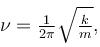where k is its force constant. What is the minimum energy of the oscillator.

Solution:

The energy of the linear harmonic oscillator is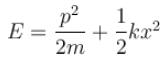This is a constant of motion. We can represent the constant value of E by means of averages of the kinetic and potential over a cycle of motion by writing.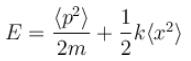The average value of x and p should vanish for an oscillating particle. So, we can identify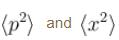with the square of the corresponding uncertainties.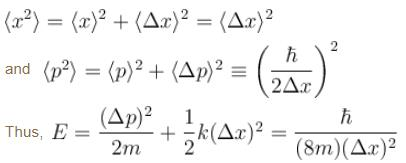Since from the uncertainty principle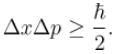To determine the minimum energy of the oscillator, we put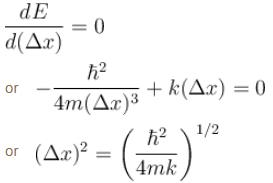The minimum energy is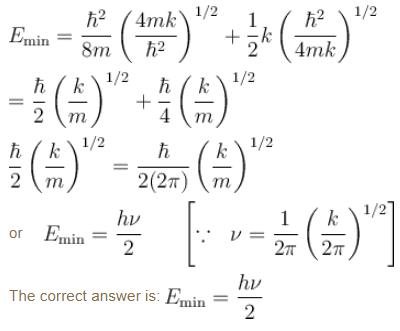QUESTION: 10

Choose the correct statement for a free particle with  ψ(x) = Aeikx

Solution: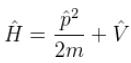V = 0 for a free particle
∴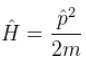Schrödinger equation.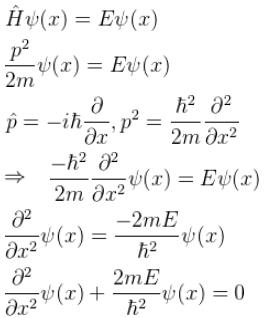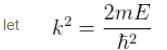∴  Aeikx and Be–ikx     (General solution)
∴  are solution to the free particle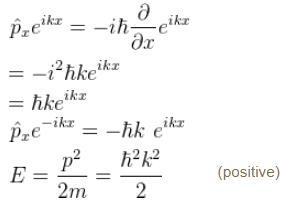The correct answer is: Energy eigenvalue is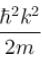and momentum is